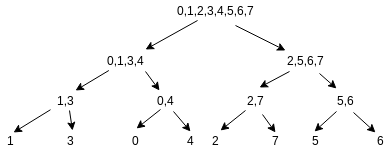# Kth smallest element in a subarray

Given an array arr of size N. The task is to find the kth smallest element in the subarray(l to r, both inclusive).

Note :

• Query are of type query(l, r, k)
• 1 <= k <= r-l+1
• There can be mutiple queries.

Examples:

Input : arr = {3, 2, 5, 4, 7, 1, 9}, query = (2, 6, 3)
Output : 4
sorted subarray in a range 2 to 6 is {1, 2, 4, 5, 7} and 3rd element is 4

Input : arr = {2, 3, 4, 1, 6, 5, 8}, query = (1, 5, 2)
Output : 2

## Recommended: Please try your approach on {IDE} first, before moving on to the solution.

```Let, S = r - l + 1.
```

Naive Approach:

• Copy the subarray into some other local array. After sorting find the kth element.

Time complexity: Slog(S)

• Use a max priority queue ‘p’ and iterate in the subarray. If size of ‘p’ is less than ‘k’ insert element else remove top element and insert the new element into ‘p’ after complete interation top of ‘p’ will be the answer.

Time complexity: Slog(k)

Efficient Approach: The idea is to use Segment trees, to be more precise use merge sort segment tree. Here, Instead of storing sorted elements we store indexes of sorted elements.

Let B is the array after sorting arr and seg is our segment tree. Node ci of seg stores the sorted order of indices of arr which are in range [st, end].

```If arr = {3, 1, 5, 2, 4, 7, 8, 6},
then B is {1, 2, 3, 4, 5, 6, 7, 8}
```

Segment tree will look like :Let’s suppose seg[ci]->left holds p elements. If p is less then or equals to k, we can find kth smallest in left child and if p is less than k then move to right child and find (k-p) smallest element.

One can find the number of elements in the sorted array(A) lying in between elements X and Y by:

upper_bound(A.begin(), A.end(), Y)-lower_bound(A.begin(), A.end(), X)

Below is the implementation of the above approach:

 `// CPP program to find the kth smallest element in a range ` `#include ` `using` `namespace` `std; ` `#define N (int)1e5 ` ` `  `// Declaring a global segment tree ` `vector<``int``> seg[N]; ` ` `  `// Function to build the merge sort ` `// segment tree of indices ` `void` `build(``int` `ci, ``int` `st, ``int` `end, ` `           ``pair<``int``, ``int``>* B) ` `{ ` `    ``if` `(st == end) { ` `        ``// Using second property of B ` `        ``seg[ci].push_back(B[st].second); ` `        ``return``; ` `    ``} ` ` `  `    ``int` `mid = (st + end) / 2; ` `    ``build(2 * ci + 1, st, mid, B); ` `    ``build(2 * ci + 2, mid + 1, end, B); ` ` `  `    ``// Inbuilt merge function ` `    ``// this takes two sorted arrays and merge ` `    ``// them into a sorted array ` `    ``merge(seg[2 * ci + 1].begin(), seg[2 * ci + 1].end(), ` `          ``seg[2 * ci + 2].begin(), seg[2 * ci + 2].end(), ` `          ``back_inserter(seg[ci])); ` `} ` ` `  `// Function to return the index of ` `// kth smallest element in range [l, r] ` `int` `query(``int` `ci, ``int` `st, ``int` `end, ` `          ``int` `l, ``int` `r, ``int` `k) ` `{ ` `    ``// Base case ` `    ``if` `(st == end) ` `        ``return` `seg[ci]; ` ` `  `    ``// Finding value of 'p' as described in article ` `    ``// seg[2*ci+1] is left node of seg[ci] ` `    ``int` `p = upper_bound(seg[2 * ci + 1].begin(), ` `                        ``seg[2 * ci + 1].end(), r) ` `            ``- lower_bound(seg[2 * ci + 1].begin(), ` `                          ``seg[2 * ci + 1].end(), l); ` ` `  `    ``int` `mid = (st + end) / 2; ` `    ``if` `(p >= k) ` `        ``return` `query(2 * ci + 1, st, mid, l, r, k); ` `    ``else` `        ``return` `query(2 * ci + 2, mid + 1, end, l, r, k - p); ` `} ` ` `  `// Driver code ` `int` `main() ` `{ ` `    ``int` `arr[] = { 3, 1, 5, 2, 4, 7, 8, 6 }; ` `    ``int` `n = ``sizeof``(arr) / ``sizeof``(arr); ` ` `  `    ``pair<``int``, ``int``> B[n]; ` ` `  `    ``for` `(``int` `i = 0; i < n; i++) { ` `        ``B[i] = { arr[i], i }; ` `    ``} ` ` `  `    ``// After sorting, B's second property is ` `    ``// something upon which we will build our Tree ` `    ``sort(B, B + n); ` ` `  `    ``// Build the tree ` `    ``build(0, 0, n - 1, B); ` ` `  `    ``cout << ``"3rd smallest element in range 3 to 7 is: "` `         ``<< arr[query(0, 0, n - 1, 2, 6, 3)] << ``"\n"``; ` `} `

Output:

```3rd smallest element in range 3 to 7 is: 5
```

Time complexity:
To build segment tree: O(n*log(n))
For each query : O(log(n)*log(n))

Attention reader! Don’t stop learning now. Get hold of all the important DSA concepts with the DSA Self Paced Course at a student-friendly price and become industry ready.

My Personal Notes arrow_drop_upCheck out this Author's contributed articles.

If you like GeeksforGeeks and would like to contribute, you can also write an article using contribute.geeksforgeeks.org or mail your article to contribute@geeksforgeeks.org. See your article appearing on the GeeksforGeeks main page and help other Geeks.

Please Improve this article if you find anything incorrect by clicking on the "Improve Article" button below.